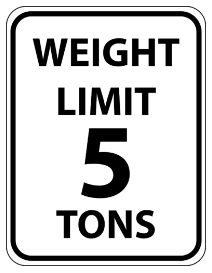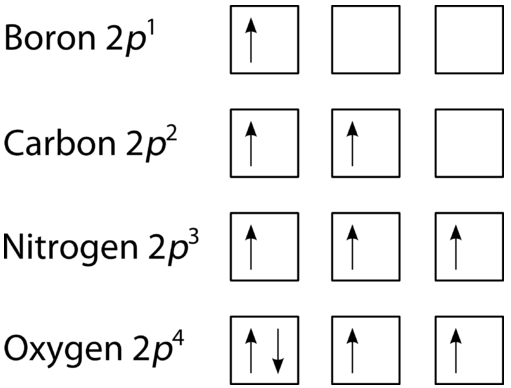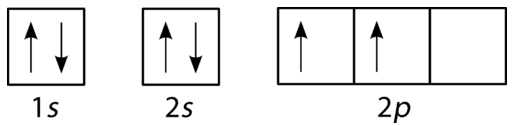# 5.19: Hund's Rule and Orbital Filling Diagrams

When placed in front of a bridge, the sign below signifies that nothing over five tons is allowed to cross, because it will do damage to the structure of the bridge. There are limits to the amount of weight that a bridge can support, there are limits to the number of people that can safely occupy a room, and there are limits to what can go into an electron orbital.## Hund's Rule

The last of the three rules for constructing electron arrangements requires electrons to be placed one at a time in a set of orbitals within the same sublevel. This minimizes the natural repulsive forces that one electron has for another. Hund's rule states that orbitals of equal energy are each occupied by one electron before any orbital is occupied by a second electron, and that each of the single electrons must have the same spin. The figure below shows how a set of three $$p$$ orbitals is filled with one, two, three, and four electrons.Figure $$\PageIndex{1}$$: The $$2p$$ sublevel, for the elements boron $$\left( Z=5 \right)$$, carbon $$\left( Z=6 \right)$$, nitrogen $$\left( Z=7 \right)$$, and oxygen $$\left( Z=8 \right)$$. According to Hund's rule, as electrons are added to a set of orbitals of equal energy, one electron enters each orbital before any orbital receives a second electron.

## Orbital Filling Diagrams

An orbital filling diagram is the more visual way to represent the arrangement of all the electrons in a particular atom. In an orbital filling diagram, the individual orbitals are shown as circles (or squares) and orbitals within a sublevel are drawn next to each other horizontally. Each sublevel is labeled by its principal energy level and sublevel. Electrons are indicated by arrows inside the circles. An arrow pointing upwards indicates one spin direction, while a downward pointing arrow indicates the other direction. The orbital filling diagrams for hydrogen, helium, and lithium are shown in the figure below.Figure $$\PageIndex{2}$$: Orbital filling diagrams for hydrogen, helium, and lithium.

According to the Aufbau process, sublevels and orbitals are filled with electrons in order of increasing energy. Since the $$s$$ sublevel consists of just one orbital, the second electron simply pairs up with the first electron as in helium. The next element is lithium and necessitates the use of the next available sublevel, the $$2s$$.

The filling diagram for carbon is shown in the figure below. There are two $$2p$$ electrons for carbon and each occupies its own $$2p$$ orbital.Figure $$\PageIndex{3}$$: Orbital filling diagram for carbon.

Oxygen has four $$2p$$ electrons. After each $$2p$$ orbital has one electron in it, the fourth electron can be placed in the first $$2p$$ orbital with a spin opposite that of the other electron in that orbital.Figure $$\PageIndex{4}$$: Orbital filling diagram for oxygen.

## Summary

• Hund's rule specifies the order of electron filling within a set of orbitals.
• Orbital filling diagrams are a way of indicating electron locales in orbitals.